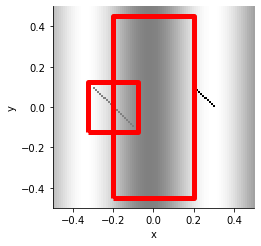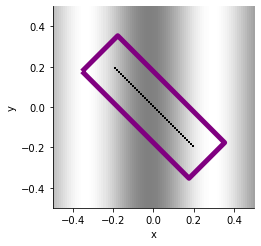# Box#

Title
Box Element
Dependencies
Matplotlib
Backends
Matplotlib
Bokeh
Plotly
```import numpy as np
import holoviews as hv
from holoviews import opts

hv.extension('matplotlib')
```A `Box` is an annotation that takes a center x-position, a center y-position and a size:

```data = np.sin(np.mgrid[0:100,0:100]/10.0)
data[np.arange(40, 60), np.arange(20, 40)] = -1
data[np.arange(40, 50), np.arange(70, 80)] = -3
(hv.Image(data) * hv.Box(-0.2, 0, 0.25 ) * hv.Box(-0, 0, (0.4,0.9))).opts(
opts.Box(color='red', linewidth=5),
opts.Image(cmap='gray'))
```In addition to these arguments, it supports an optional `aspect ratio`:

By default, the size argument results in a square such as the small square shown above. Alternatively, the size can be given as the tuple `(width, height)` resulting in a rectangle. If you only supply a size value, you can still specify a rectangle by specifying an optional aspect value. In addition, you can also set the orientation (in radians, rotating anticlockwise):

```data = np.sin(np.mgrid[0:100,0:100]/10.0)
data[np.arange(30, 70), np.arange(30, 70)] = -3
box = hv.Box(-0, 0, 0.25, aspect=3, orientation=-np.pi/4)

(hv.Image(data) * box).opts(
opts.Box(color='purple', linewidth=5),
opts.Image(cmap='gray'))
```For full documentation and the available style and plot options, use `hv.help(hv.Box).`

This web page was generated from a Jupyter notebook and not all interactivity will work on this website. Right click to download and run locally for full Python-backed interactivity.